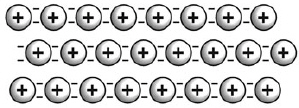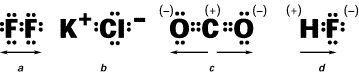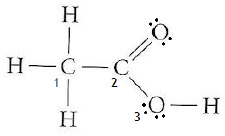Name:    Bonding Quiz Practice

Matching

Match each item with the correct statement below.
 a. metallic bond c. valence electron b. octet rule d. electron dot structure

1.

an electron in the highest occupied energy level of an atom

2.

Atoms react so as to acquire the stable electron structure of a noble gas.

3.

a depiction of valence electrons around the symbol of an element

4.

the attraction of valence electrons for metal ions

Multiple Choice
Identify the choice that best completes the statement or answers the question.

5.

The rule that sates that elements will gain, lose or share electrons in order to have a full outer shell is called?
 a. None of these b. Foster’s rule c. Octet rule d. The Rule of 2

6.

How many electrons are needed in the outer energy levels of most atoms for the atom to be chemically stable?
 a. 8 b. 6 c. 4 d. 2

7.

What will happen to Oxygen, O when it bonds?
 a. lose 2 protons b. gain 2 electrons c. lose 2 electrons d. lose 6 electrons

8.

What will happen to Magnesium , Mg when it bonds?
 a. gain 6 electrons b. gain 2 protons c. gain 2 electrons d. lose 2 electrons

9.

Why do the noble gases NOT form compounds readily?
 a. They have no electrons. b. They have empty outer energy levels. c. Their outer energy levels are completely filled with electrons. d. They have seven electrons in the outer energy levels.

10.

What kind of chemical bond is formed when a transfer of electrons occurs?
 a. ionic b. hydrate c. magnetic d. covalent

11.

A chemical bond that occurs when atoms share electrons is a(n) ____ bond.
 a. polyatomic b. ionic c. covalent d. magnetic

12.

Ionic compounds are held together by a force best compared to________________?
 a. magnetic attraction c. both a and b b. electronic repulsion d. neither a or b

13.

A double covalent bond contains _____  electrons?
 a. 6 b. 0 c. 2 d. 4

14.

Bonds that form between two nonmetals are usually
 a. impossible. b. weak. c. ionic. d. covalent.

15.

In an electron dot diagram, the dots are used to represent
 a. neutrons c. all electrons. b. valence electrons. d. protons

16.

The formation of an ionic bond involves the
 a. sharing of electrons. c. transfer of electrons. b. transfer of protons. d. transfer of neutrons.

17.

A chemical bond that occurs when atoms share electrons UNEQUALLY is a(n) ____ bond.
 a. polar covalent c. nonpolar covalent b. polar ionic d. polyatomic

18.

A chemical bond that occurs when atoms share electrons EQUALLY is a(n) ____ bond.
 a. polyatomic b. polar c. nonpolar d. ionic

19.

Which is true of the model of bonding shown in this figure?a. Metallic atoms are present in a “sea” of negatively charged atoms. b. Valence electrons are able to move easily among the metallic cations. c. It results in the substance being very brittle and not easily deformed. d. Heat and electricity are easily carried by the cations from one region to another.

20.

How many valence electrons are in an atom of phosphorus?
 a. 2 b. 3 c. 4 d. 5

21.

How many valence electrons are in an atom of magnesium?
 a. 2 b. 3 c. 4 d. 5

22.

How many valence electrons are in a silicon atom?
 a. 2 b. 4 c. 6 d. 8

23.

How does oxygen obey the octet rule when reacting to form compounds?
 a. It gains electrons. b. It gives up electrons. c. It does not change its number of electrons. d. Oxygen does not obey the octet rule.

24.

What characteristic of metals makes them good electrical conductors?
 a. They have mobile valence electrons. b. They have mobile protons. c. They have mobile cations. d. Their crystal structures can be rearranged easily.

25.

How many single covalent bonds can carbon form?
 a. 1 b. 2 c. 3 d. 4

26.

How many single covalent bonds can halogens form?
 a. 1 b. 2 c. 3 d. 4

27.

In the compound PCl3, how many total valence electrons are present?
 a. 4 b. 8 c. 24 d. 26

28.

Which type of bond has an electronegativity difference greater than 1.7?
 a. ionic c. nonpolar covalent b. metallic d. polar covalent

29.

What molecular shape does the compound CCl4 have?
 a. trigonal planar c. octahedral b. tetrahedral d. bent

30.

Fluorine belongs to group 7A / 17. How many covalent bonds are formed between two fluorine atoms?
 a. 1 b. 2 c. 3 d. 4

31.

If the electronegativity of H is 2.20 and of Cl is 3.55, which type of bond is formed between H and Cl, when they form hydrogen chloride?
 a. Ionic c. Covalent b. Polar ionic d. Polar covalent

32.

Which of the following contains a double bond?a. a b. b c. c d. d

33.

Which of the following COVALENT compounds is the most polar?a. a b. b c. c d. d

34.

Which of the following central atoms has a 2:0 ratio and has a linear shape?a. 1 c. 3 b. 2 d. none of these